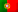Home > Technology > Computer Science > Programming > C++ > Dynamic arrays with structs in C++

# Dynamic arrays with structs in C++

In previous posts (“Using pointers in C / C++” and “malloc and realloc functions in C / C++“) I talked about pointers and dynamic arrays in C/C++. On this article I’m going to continue talking about this subject and making an approach on handling arrays for a type “struct”.

In C++, a “struct” is like a class but without methods, just properties (variables). Another difference from classes, is that in a “struct”, properties are public by default. Now, imagine that we want to create a list of contacts where we can insert a name, a phone number and an e-mail address. We can define our “struct” as:

```struct Contacts {
char name;
char phone;
char email;
};
```

We also want our list of contacts to have a dynamic capacity and that it won’t be limited to a fixed number of inputs. We saw, on the article “malloc and realloc functions in C / C++“, how to use the “malloc” and “realloc” functions to build a dynamic array of integers. We also saw some solutions on how to handle the array’s capacity and I used there the second solution, where we started with a capacity for 10 elements and then we increased that capacity by 10, whenever we reached its limit. On this article, I’m going to use the third solution and, in the following example, we will start the array with a capacity for 5 elements and double that capacity whenever we reached its limit.

The following code shows how to initialize our variables:

```    int index = 0;
int capacity = 5;
Contacts *contacts = NULL;

contacts = (Contacts *) malloc(sizeof(Contacts)*capacity);
```

Notice that the code is very similar to the one we saw in the previous post. It is important to have in mind that, when we use the function “sizeof” and dynamic arrays, our structure should have a fixed size! For that, I used arrays of chars with a fixed size for the contact’s fields. Otherwise, it wouldn’t be possible to make a direct access to an element “contacts[i]” because the compiler wouldn’t know how to determine its exact position, as I also explained in the previous post.

In order to insert element and manage the array’s capacity, we have the following code:

```    Contacts c;
cout << "Name: ";
cin >> c.name;
cout << "Phone: ";
cin >> c.phone;
cout << "E-Mail: ";
cin >> c.email;
contacts[index] = c;
index++;
if (index>=capacity) {
capacity*=2;
contacts = (Contacts *) realloc(contacts, sizeof(Contacts)*capacity);
}
```

Notice that, in this case, we are doubling the capacity of the array each time we reach its limit (line 11). I would like to add that we could always use the function “realloc”. When we first initialize the array “contacts” with the function “malloc”, we could also do it as follows:

```        contacts = (Contacts *) realloc(contacts, sizeof(Contacts)*capacity);
```

Just notice that the array was declared and initialized as “NULL”. When “realloc” receives a NULL pointer, it will create a new allocation.

Now, let’s take a look in the full program:

```#include <iostream>
#include <stdlib.h>

using namespace std;

struct Contacts {
char name;
char phone;
char email;
};

int main() {

int index = 0;
int capacity = 5;
Contacts *contacts = NULL;

contacts = (Contacts *) malloc(sizeof(Contacts)*capacity);

cout << "1 - Insert contact" << endl;
cout << "2 - List contacts" << endl;
cout << "3 - Array state" << endl;
cout << "0 - Exit program" << endl;

case '0':
break;
case '1':
Contacts c;
cout << "Name: ";
cin >> c.name;
cout << "Phone: ";
cin >> c.phone;
cout << "E-Mail: ";
cin >> c.email;
contacts[index] = c;
index++;
if (index>=capacity) {
capacity*=2;
contacts = (Contacts *) realloc(contacts, sizeof(Contacts)*capacity);
}
break;
case '2':
for (int i=0; i<index; i++) {
cout << "Input " << i << ":" << endl;
cout << "  Name: " << contacts[i].name << endl;
cout << "  Phone: " << contacts[i].phone << endl;
cout << "  E-Mail: " << contacts[i].email << endl;
}
break;
case '3':
cout << "Inserted elements: " << index << endl;
cout << "Array capacity: " << capacity << endl;
break;
default:
printf("Invalid option!");
break;
}

}

free(contacts);

return 0;

}
```

Some considerations and differences on this code comparing with the one in the previous post:

• This implementation is focused in C++ and I used the library “iostream” instead of “stdio.h” (line 1) and to make things easier to use the “cout” and “cin” statements, I used the “namespace std” (line 4)
• When using integers, “cin” may have some undesirable behaviours (line 27), so the variable “menu” was declared as a char (line 14). Obviously, there are ways to to workaround that problem and we could have also used the “fgets” function instead. However, the goal here is to simplify the code and focus on what really matters.
• On line 33, it was declared an instance of “Contacts” to receive the user inputs. We can store the user inputs directly in the array but I personally don’t consider that a good practice, specially if we want to validate the data before copying it into the array.

Follow us on the social networks and stay tuned with news from our multi-thematic blog – Out4Mind!